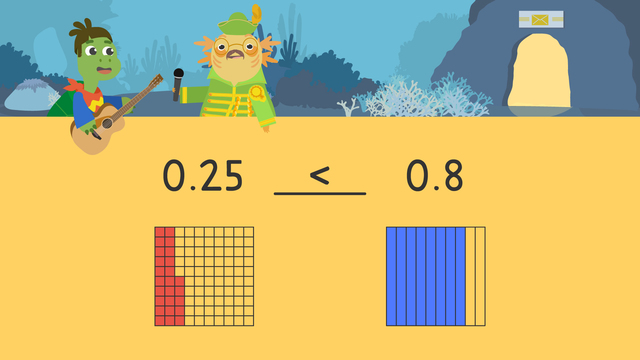# Comparing Decimals: Tenths and HundredthsRating

Ø 5.0 / 1 ratings

The authorsTeam Digital
Comparing Decimals: Tenths and Hundredths
CCSS.MATH.CONTENT.4.NF.C.7

## Basics on the topicComparing Decimals: Tenths and Hundredths

### In This Comparing Decimals Video

Axel and Tank are sorting boxes at their new job. In order to sort them, they have to practice comparing decimal tenth and hundredth based on the number on the box’s label and put them in a greater than, less than, or equal to bin. Let’s help Axel and Tank get their work done by comparing decimal tenth and hundredth!

### Comparing Decimal Tenth and Hundredth

When comparing decimals tenths and hundredths, use the greater than, less than, or equal to symbols.In this comparing decimals video, we practice comparing decimals with models in addition to looking at place value, because base ten blocks help us visualize numbers. Let’s take a look at a comparing decimals example.

### Comparing Decimals Example

The first labels they have to compare are twenty-five hundredths and eight tenths. Below, we have twenty-five out of one hundred squares shaded in, and eight out of ten strips shaded in.When comparing decimals you need to start with the greatest place value, the ones place. Since the zeros are equal, we move to the next place value, the tenths place. Twenty-five hundredths has a two in the tenths place, and eight tenths has an eight in the tenths place.Since we found a digit greater or less than, we can stop comparing! Twenty-five hundredths is less than eight tenths.### Comparing Decimal Tenth and Hundredth Summary

Remember, when comparing decimals: